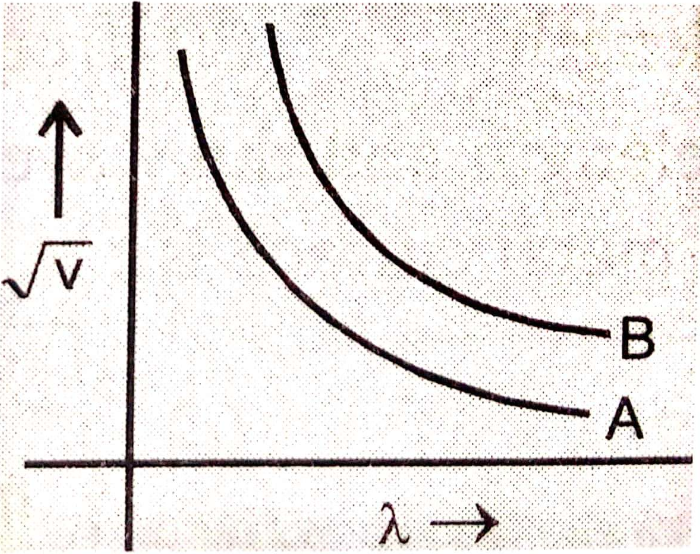The de Broglie wavelength of electron of He+ ion is 3.329Å. If the photon emitted upon de-excitation of this He+ ion is made to hit H atom in its ground state so as to liberate electron from it, what will be the de Broglie's wavelength of photoelectron ?

(A) 0.1518 Å

(B) 6.1518 Å

(C) 2.3518 Å

(D) 4.1218 Å

Concept Questions :-

de Broglie Equation
High Yielding Test Series + Question Bank - NEET 2020

Difficulty Level:

Calculate the de Broglie wavelength of an $\alpha$-particle emitted from radium having an energy of 4.8 MeV.

(Mass of $\alpha$-particle=6.6 x 10-24 g; h=Planck's constant = 6.626 x 10-27 erg sec)

(A) 6.18 x 10-13cm

(B) 0.58 x 10-8cm

(C) 6.58 x 10-13cm

(D) 4.12 x 10-11cm

Concept Questions :-

de Broglie Equation
High Yielding Test Series + Question Bank - NEET 2020

Difficulty Level:

What is the ratio of the time period of revolution of electron He+ ion sample in the orbit where the path length is three time the de-broglies wavelength to the time period of revolution in the ground state –

(A) 27:1

(B) 1:27

(C) 9:8

(D) 8:9

Concept Questions :-

de Broglie Equation
High Yielding Test Series + Question Bank - NEET 2020

Difficulty Level:

For particles having same kinetic energy, the de Broglie wavelength is-

(A) Directly proportional to its velocity

(B) Inversely proportional to its velocity

(C) Independent of velocity and mass

(D) Unpredictable

Concept Questions :-

de Broglie Equation
High Yielding Test Series + Question Bank - NEET 2020

Difficulty Level:

An electron, practically at rest, is initially accelerated through a potential difference of 100 volts. It then has a de Broglie wavelength=$\lambda$1Å. It then get retarded through 19 volts and then it has wavelength $\lambda$2 Å. A further retardation through 32 volts changes the wavelength to $\lambda$3.

What is ($\lambda$3-$\lambda$2)/$\lambda$1?

(A) 20/41

(B) 10/63

(C) 20/63

(D) 10/41

Concept Questions :-

de Broglie Equation
High Yielding Test Series + Question Bank - NEET 2020

Difficulty Level:

A cricket ball of 0.5 kg is moving with a velocity of 100 ms-1. The wavelength associated with its motion is:

1. 1/100cm

2. 6.6 x 10-34m

3. 1.32 x 10-35m

4. 6.6 x 10-28m

Concept Questions :-

de Broglie Equation
High Yielding Test Series + Question Bank - NEET 2020

Difficulty Level:

If the velocity of a particle is reduced to 1/3rd, then percentage increase in its de-broglie wavelength will be:-

1. 100%

2. 200%

3. 50%

4. 33.33%

Concept Questions :-

de Broglie Equation
High Yielding Test Series + Question Bank - NEET 2020

Difficulty Level:

Which of the following has the largest de Broglie wavelength (all have equal velocity)

1.  ${\mathrm{CO}}_{2}$ molecule

2.  ${\mathrm{NH}}_{3}$ molecule

3.  Electron

4.  Proton

Concept Questions :-

de Broglie Equation
High Yielding Test Series + Question Bank - NEET 2020

Difficulty Level:

$\sqrt{\mathrm{V}}$on two particles A and B are plotted against de-Broglie wavelengths. Where V is the potential on the particles. Which of the following relation is correct about the mass of particles?1.  ${\mathrm{m}}_{\mathrm{A}}={\mathrm{m}}_{\mathrm{B}}$

2.  ${\mathrm{m}}_{\mathrm{A}}>{\mathrm{m}}_{\mathrm{B}}$

3.  ${\mathrm{m}}_{\mathrm{A}}<{\mathrm{m}}_{\mathrm{B}}$

4.  ${\mathrm{m}}_{\mathrm{A}}\le {\mathrm{m}}_{\mathrm{B}}$

Concept Questions :-

de Broglie Equation